Published

# Event Counter

Arduino counter of pulses with preset.

IntermediateFull instructions provided634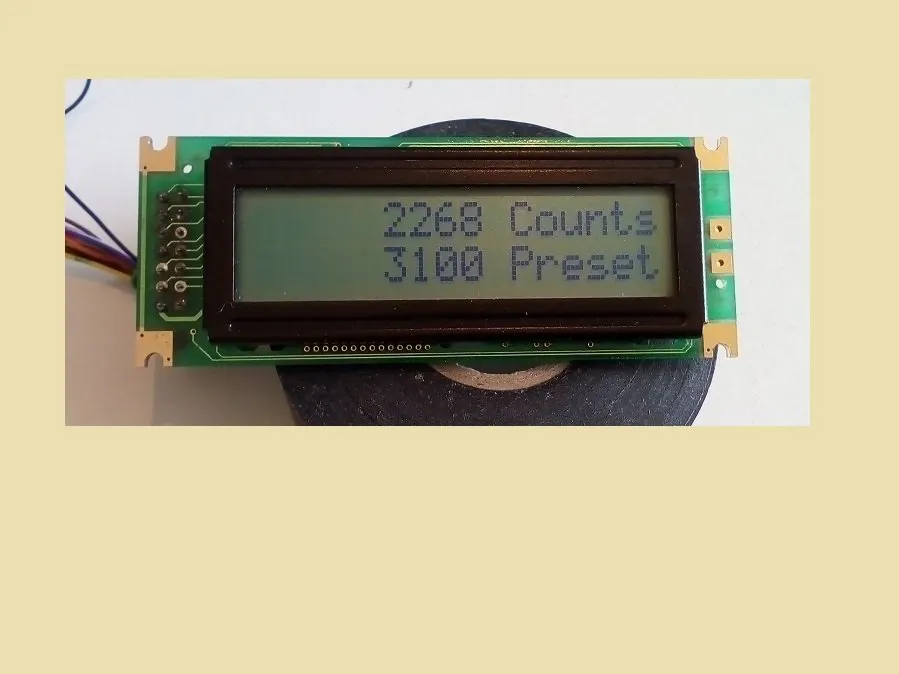## Things used in this project

### Hardware componentsArduino Nano R3
×1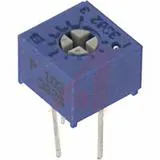Single Turn Potentiometer- 10k ohms
×1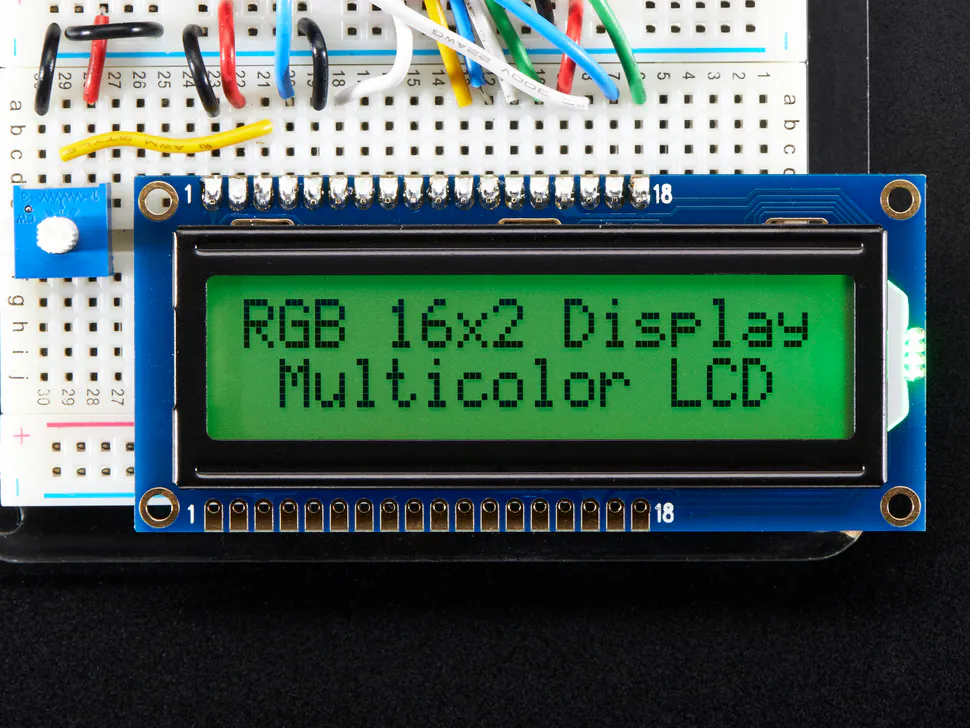Adafruit RGB Backlight LCD - 16x2
×1SparkFun Pushbutton switch 12mm
×2

## Schematics

### counter2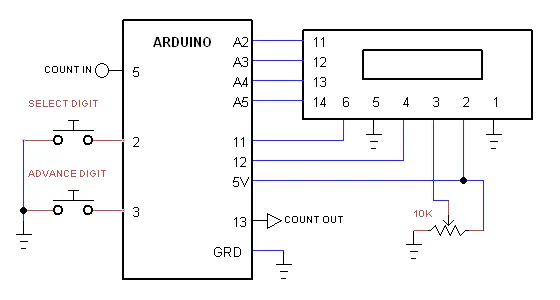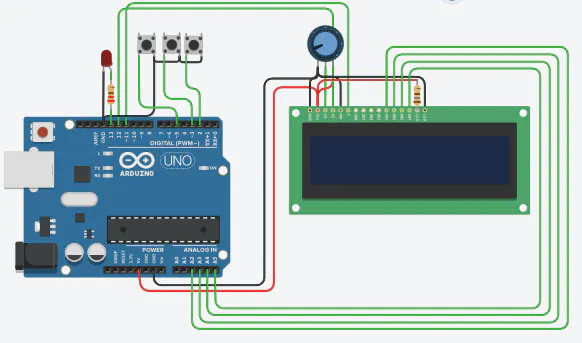## Code

### event_counter

Arduino
```/*
* Event Counter
* Created: 4/5/20
*  Author: moty22.co.uk
*/

#include <LiquidCrystal.h>

unsigned char d, set=0;
unsigned long count=0, preset=0;

// initialize the library with the numbers of the interface pins
LiquidCrystal lcd(12, 11, A2, A3, A4, A5);

void setup() {

lcd.begin(16, 2);   // set up the LCD's number of columns and rows:
pinMode(2, INPUT_PULLUP);
pinMode(3, INPUT_PULLUP);
pinMode(5, INPUT_PULLUP);
pinMode(13, OUTPUT);    //counts reached preset output
digitalWrite(13,LOW);

TCCR1B=0b111;  //  input T1 pin D5
TCNT1 = 0;

}

void loop() {

for(byte i=0;i<50;++i){
delay(10);
count += (unsigned long)TCNT1;    //total counts = prev + new counts
TCNT1 = 0;  //reset timer1
if(count >= preset) {digitalWrite(13,HIGH);} else{digitalWrite(13,LOW);}
}
display();

set +=1;
if(set==7){set=0; lcd.noCursor();}
display();

}
//setting 6 digits of preset
while(set>0){
lcd.setCursor(8-set, 1);
lcd.cursor();

d[set] +=1;
if(d[set]>9){d[set]=0;}
preset = (unsigned long)d + (unsigned long)d*10 + (unsigned long)d*100 + (unsigned long)d*1000 +
(unsigned long)d*10000 + (unsigned long)d*100000; //calculate preset
delay(500);
display();
}
}

}

void display(){

String str_count = String(count, DEC);  //convert counts to a string
String str_preset = String(preset, DEC);  //convert preset to a string
lcd.clear();
lcd.setCursor(8 - str_count.length(), 0);  // align string to right
lcd.print(count, DEC);
lcd.setCursor(10, 0);
lcd.print("Counts");
lcd.setCursor(8 - str_preset.length(), 1);  // align string to right
lcd.print(preset, DEC);
lcd.setCursor(10, 1);
lcd.print("Preset");
//   delay(200);
}
```